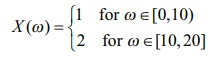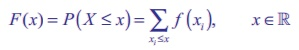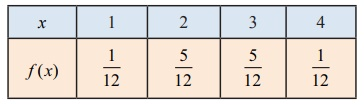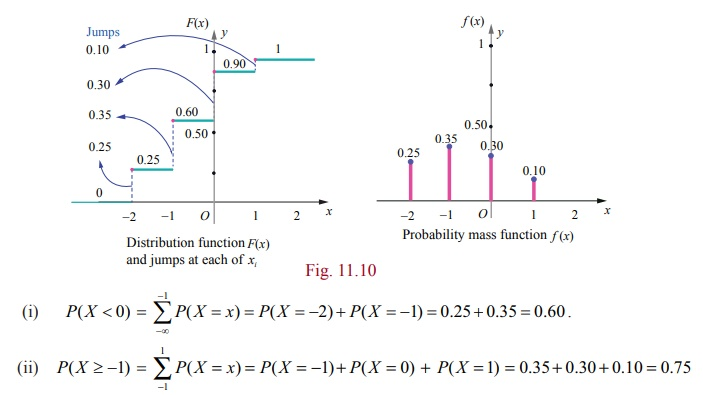Home | | Maths 12th Std | Types of Random Variable

# Types of Random Variable

(i) Discrete Random variable (for counting the quantity) (ii) Continuous Random variable (for measuring the quantity)

Types of Random Variable

In this chapter we shall restrict our study to two types of random variables, one is a random variable assuming at most a countable number of values and another is a random variable assuming the values continuously. That is

(i) Discrete Random variable (for counting the quantity)

(ii) Continuous Random variable (for measuring the quantity)

## 1. Discrete random variables

In this section we discuss

(i) Discrete random variables

(ii) Probability mass function

(iii) Cumulative distribution function.

(iv) Obtaining cumulative distribution function from probability mass function.

(v) Obtaining probability mass function from cumulative distribution function.

If the range set of the random variables is discrete set of numbers then the inverse image of random variable is either finite or countably infinite. Such a random variable is called discrete random variable. A random variable defined on a discrete sample space is discrete.

### Definition 11.2 (Discrete Random Variable)

A random variable X is defined on a sample space S into the real numbers is called discrete random variable if the range of X is countable, that is, it can assume only a finite or countably infinite number of values, where every value in the set S has positive probability with total one.

Remark

It is also possible to define a discrete random variable on continuous sample space. For instance,

(i) for a continuous sample space S = [0,1] , the random variable defined by X (ω) = 10 for all ω S, is a discrete random variable.

(ii) for a continuous sample space S = [0, 20] , the random variable defined byis a discrete random variable.

## 2. Probability Mass Function

The probability that a discrete random variable X takes on a particular value x, that is P ( X = x) , is frequently denoted by f ( x) or p ( x) . The function f ( x) is typically called the probability mass function, although some authors also refer to it as the probability function or the frequency function. In this chapter, when the random variable is discrete, the common terminology the probability mass function is used and its common abbreviation is pmf.

### Definition 11.3 (Probability mass function)

If X is a discrete random variable with discrete values x1 , x2 , x3 , xn , then the function denoted by f (.) or p(.) and defined by

f ( xk ) = P ( X= xk ), for k =1, 2, 3, n,

is called the probability mass function of X

### Theorem 11.1 (Without proof)

The function f ( x) is a probability mass function if and only if it satisfies the following properties for the set of real values x1, x2, x3, ... xn ....

(i) f (xk) 0 for k =1, 2, 3, n, and

(ii) ∑k f (xk) =1

Note:

(i) The set of probabilities { f (xk ) = P ( X = xk ), k = 1, 2, 3,. . . . n , . . . } is also known as probability distribution of discrete random variable

(ii) Since the random variable is a function, it can be presented

(a) in tabular form (b) in graphical form and (c) in an expression form

Example 11.5

Two fair coins are tossed simultaneously (equivalent to a fair coin is tossed twice). Find the probability mass function for number of heads occurred.

Solution

The sample space S = { H , T} × {H , T}

That is S = { TT , TH, HT , HH }

Let X be the random variable denoting the number of heads.

Therefore

X (TT) =0,

X (TH) =1,

X (HT) = 1, and

X (HH) =2.

Then the random variable X takes on the values 0, 1 and 2The probabilities are given byTherefore f ( x) is a probability mass function.

The probability mass function is given byExample 11.6

A pair of fair dice is rolled once. Find the probability mass function to get the number of fours.

Solution

Let X be a random variable whose values x are the number of fours.The sample space S is given in the table.

It can also be written as

S = {(i, j)} , where i = 1, 2, 3,. . .6 and j = 1, 2, 3, 6

Therefore X takes on the values of 0, 1, and 2.

We observe that

(i) X = 0, if (i , j) for i 4, j 4,

(ii) X = 1, if (1,4) , (2,4) , (3,4) , (5,4) , (6,4) , (4,1) , (4, 2) , (4, 3) , (4, 5) , (4, 6)

(iii) X = 2, if (4, 4) ,

Therefore,## 3. Cumulative Distribution Function or Distribution Function

There are many situations to compute the probability that the observed value of a random variable X will be less than or equal to some real number x . Writing F( x) = P (X≤ x) for every real number x , we call F ( x) , the cumulative distribution function or distribution function of the random variable X and its common abbreviation is cdf .

### Definition 11.4: (cumulative distribution function)

The cumulative distribution function F ( x) of a discrete random variable X , taking the values x1 , x2 , x3 , such that x1 < x2 < x3 <with probability mass function f (xi) is

F ( x) = P ( X x ) = xi≤x f ( xi ), x ∈ ℝThe distribution function of a discrete random variable is known as Discrete Distribution Function. Although, the probability mass function f ( x) is defined only for a set of discrete values x1, x2 , x3 , . . . , the cumulative distribution function F ( x) is defined for all real values of x .

We can compute the cumulative distribution function using the probability mass functionIf X takes only a finite number of values x1 , x2 , x3 , . . . xn , where x1 < x2 < x3 <, . . .  < xn , then the cumulative distribution function is given byFor a discrete random variable X, the cumulative distribution function satisfies the following properties.

(i) 0 F (x) 1 , for all x .

(ii) F ( x) is real valued non-decreasing function { x < y, then F ( x) ≤ F ( y) .

(iii) F ( x) is right continuous function (limx→a+ F(x) =  F (a) .

(iv) lim x → -∞ F (x) = F(-∞) = 0 .

(v) lim x → +∞ F (x) = F(+∞) = 1 .

(vi) P (x1 < X ≤  x2 ) = F (x2) − F (x1).

(vii) P (X > x) = 1 − P (X≤ x) = 1 − F (x) .

(viii) P ( X = xk ) = F( xk ) F( xk ) .

Note

Some authors use left continuity in the definition of a cumulative distribution function F ( x) , instead of right continuity.

## 4. Cumulative Distribution Function from Probability Mass function

Both the probability mass function and the cumulative distribution function of a discrete random variable X contain all the probabilistic information of X. The probability distribution of X is determined by either of them. In fact, the distribution function F of a discrete random variable X can be expressed in terms of the probability mass function f(x) of X and vice versa.

### Example 11.7

If the probability mass function f ( x) of a random variable X isfind (i) its cumulative distribution function, hence find (ii) P( X 3) and, (iii) P( X 2)

### Solution

(i) By definition the cumulative distribution function for discrete random variable is

F ( x) = P( X≤ x) = ∑xi≤xP( X = xi )Therefore the cumulative distribution function is### Example 11.8

A six sided die is marked ‘1’ on one face, ‘2’ on two of its faces, and ‘3’ on remaining three faces. The die is rolled twice. If X denotes the total score in two throws.(i) Find the probability mass function.

(ii) Find the cumulative distribution function.

(iii) Find P (3≤ X < 6) (iv) Find P ( X 4) .

Solution:

Since X denotes the total score in two throws, it takes on the values 2, 3, 4, 5, and 6.

From the Sample space S, we have## 5. Probability Mass Function from Cumulative Distribution Function

For a discrete random variable X, the cumulative distribution function F has jumps at each of the xi , and is constant between successive xi s . The height of the jump at xi f( xi ) ; in this way the is probability at xi can be retrieved from F .

Suppose X is a discrete random variable taking the values x1 , x2 , x3 , such that x1 < x2 , < x3 and F ( xi ) is the distribution function. Then the probability mass function f ( xi ) is given by

f (xi) = F (xi ) F (xi-1 ) , i = 1, 2, 3, . . .

Note

The jump of a function F ( x) at x = a is |F ( a + ) F ( a )|. Since F is non-decreasing and continuous to the right, the jump of a cumulative distribution function F is P( X = x) = F( x) − F( x ) .

Here the jump (because of discontinuity) acts as a probability. That is, the set of discontinuities of a cumulative distribution function is at most countable!

### Example 11.9

Find the probability mass function f ( x) of the discrete random variable X whose cumulative distribution function F ( x) is given byAlso find (i) P( X < 0) and (ii) P( X ≥ − 1) .

### Solution

Since X is a discrete random variable, from the given data, X takes on the values 2,1, 0, and 1.

For discrete random variable X, by definition, we have f ( x) = P( X = x)

Therefore left hand limit of F(x) at x = −2 is F ( 2 )

f(2) = P(X = − 2) = F(− 2) −  F(− 2  ) = 0.25 − 0 = 0.25.

Similarly for other jump points, we have

f (1) = P(X = − 1) = F(− 1) −  F(−2) = 0.60 − 0.25 = 0.35.

f (0) = P(X = 0) = F(0) −  F(−1) = 0.90 −  0.60 = 0.30 ,

f (1) = P( X = 1) = F(1)F(0) = 1 −  0.90 = 0.10 .

Therefore the probability mass function isThe distribution function F ( x) has jumps at x = − 2, − 1, 0, and 1. The jumps are respectively 0.25, 0.35, 0.30, and 0.1 is shown in the figure given below.

These jumps determine the probability mass functionExample 11.10

A random variable X has the following probability mass function.Find (i) P (2 < X < 6) (ii) P (2 ≤ X < 5) (iii) P(X 4) (iv) P(3< X)

Solution

Since the given function is a probability mass function, the total probability is one. That is x f (x) = 1.

From the given data k + 2k + 6k + 5k + 6k + 10k = 1

30k = 1

k = 1/30

Therefore the probability mass function isTags : Probability Distributions | Mathematics , 12th Maths : UNIT 11 : Probability Distributions
Study Material, Lecturing Notes, Assignment, Reference, Wiki description explanation, brief detail
12th Maths : UNIT 11 : Probability Distributions : Types of Random Variable | Probability Distributions | Mathematics